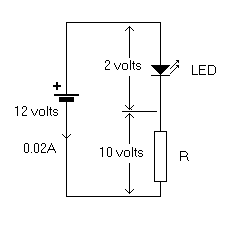Electronic Circuits and Tutorials
Tutorials
Dictionaries
Projects
Discover
Home > Electronics Tutorial > The Diode Tutorial > Using the Light Emitting Diode Tutorial
The Diode - Electronic Tutorials

# Using the Light Emitting Diode TutorialThe light emitting diode (LED) is commonly used as an indicator. It can show when the power is on, act as a warning indicator, or be part of trendy jewelry etc. It needs to be fed from a DC supply, with the anode positive and the cathode negative, as shown in the diagram.

To calculate the value of the series resistor we need to know the diode forward voltage and current and its connections. The necessary data can be obtained from a catalogue or data book. In our example it is 2 volts and 20mA (0.02 amps). The cathode lead is the one nearest a "flat" on the body.

Since the voltage across the diode is 2 volts and the battery voltage is 12 volts, then the voltage across the resistor is 12-2 = 10 volts. The diode is in series with the resistor, so the current through then both is the same, 0.02 amps.

We now know the voltage across, and the current through the resistor. From Ohm's Law we can now calculate the value of the resistor.

Resistance = Volts divided by Amps = V/I = 10/0.02 =500 ohms.

Since this is not a standard value we can use a 470 or 560 ohm resistor as this application is not critical of values.

Note: To report broken links or to submit your projects, tutorials please email to Webmaster